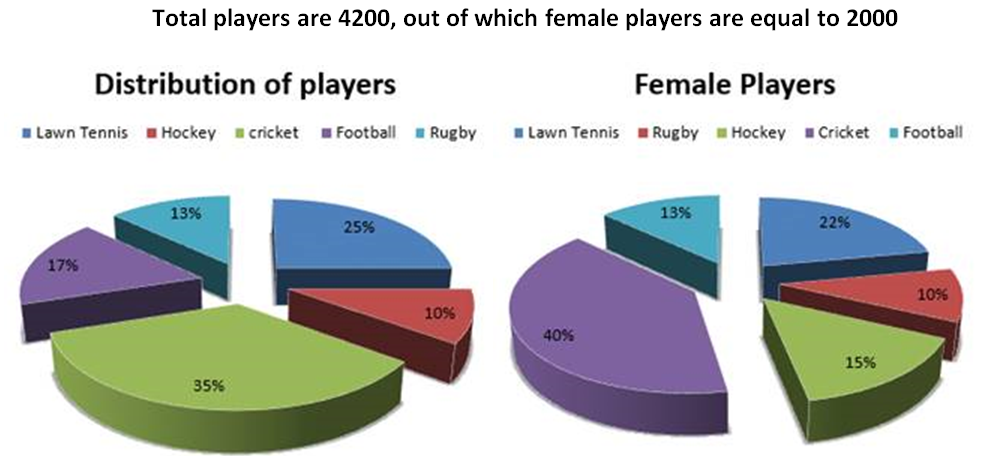Data Interpretation Quiz for SBI PO 2017

Directions (1 – 5): Study the following pie-chart carefully to answer these questions: Percentage wise distribution of players who play five different sports.1. What is the average number of players (both male and female) who play Football and Rugby together?
a) 620
b) 357
c) 230
d) 630
e) None of these

2. What is the difference between the number of female players who play Lawn Tennis and the number of male players who play Rugby?
a) 94
b) 84
c) 220
d) 240
e) None of these
3. What is the ratio of the number of female players who play Cricket to the number of male players who play Hockey?
a) 20 : 7
b) 4 : 21
c) 20 : 3
d) 3 : 20
e) None of these
4. What is the ratio of the number of female players who play Cricket to the number of male players who play Hockey?
a) 1,724
b) 1,734
c) 1,824
d) 1,964
e) None of these
5. The number of male players who play Rugby is approximately what percentage of the total number of players who play Lawn Tennis?
a) 23
b) 39
c) 26
d) 21
e) 43
Directions (6 – 10): Refer to the table and answer the following questions.
The table shows the performance of 6 batsmen.
 Name of Batsman Number of matches played by batsman in the tournament Average run scored by batsman in the tournament Total balls faced in the tournament Strike Rate Virat 20 – – 160 Rohit 16 55 – – Rahane – 60 400 120 Dhoni – – – 80 Shikhar 10 70 800 – Pujara – – – 70
(i) Strike Rate= (Total Runs Scored/Total balls faced)*100
(ii) All the batsman could bat in all the matches played
(iii) You have to calculate the missing value and give the answer accordingly.
6. If the respective ratio between the balls faced by Dhoni and Pujara is 4:5, then by what percent did Pujara score more than Dhoni?
a) 5.27%
b) 9.67%
c) 8.57%
d) 9.37%
e) Cannot be determined
7. If the runs scored by Shikhar in the last 3 matches of the tournament are not considered, his average decreases by 15. If the runs scored by Shikhar in 8th and 9th match are below 100 and no two scores among these 3 scores are equal, what is the minimum possible run scored by Shikhar in the 10th match?
a) 115
b) 116
c) 117
d) 118
e) Cannot be determined
8. Total balls faced by Virat is 600 less than the total runs made by him. What is the average of Virat in the tournament?
a) 70
b) 75
c) 80
d) 85
e) Cannot be determined
9. Rohit faces equal number of balls in first 8 matches he played in the tournament and the last 8 matches he played in the tournament. If his strike rate in first 8 and last 8 matches of the tournament are 80 and 96 respectively, What is the total number of balls faced by him in the tournament?
a) 500
b) 400
c) 1000
d) 800
e) 1200
10. What is the number of match played by Rahane in the tournament?
a) 10
b) 12
c) 8
d) 6
e) 9
Solutions:
1. D) avg. no. of players who play football and rugby together =1/2 × 30% of 4200 = 630
2. A) number of female players who play lawn tennis = 22% of 2000= 440 ,
Number of male players who play rugby = 13% of 4200 – 10% of 2000 = 346,
Reqd. = 440 – 346 = 94
3. C) number of players who do not play cricket = 40% of 2000= 800
Number of male players who play hockey = 10% of 4200 – 15% of 2000 = 420 – 300 = 120 ,
reqd. ratio = 800 : 120 = 20 : 3
4. B) reqd. number of male players who play football, cricket and lawn tennis together = 77% of 4200 – 75% of 2000 = 3234 – 1500 = 1734
5. A) Number of male who play ruby = 13% of 4200 – 10% of 2000 = 546 – 200 = 346
Total number of players who play Lawn Tennis = 25% of 4200 = 1500
Required percentage = 346/1500 × 100 = 23.06 ≈ 23%
6. D) Strike rate = (Run/Ball)*100=> Run= (S.R * Ball)/100
Let ball Dhoni:Pujara =4x:5x
Run by Dhoni(D)=80*4x/100=32x/10
Run by Pujara=70*5x/100=35x/10
Required %=(35x-32x)/32x *100=9.375%
7. D) Total runs scored by Shikhar in 10 matches = 10*70= 700
Runs scored in 1st seven matches =7*(70-15)=385 (as avg reduces by 15)
Runs scored in last 3 matches=700-385=315(8th , 9th and 10th match)
Minimum run in 10th match means maximum runs in 8th and 9th match.
But run in 8th and 9th matches are below 100, => one is 99 other is 98 (as no run is equal)
Hence run in 10th match=315-(99+98)=118
8. C) Let average=x =>Total run=20x
Balls=20x -600
=>(20x/(20x-600))*100=160 [Strike Rate]
Solve x=80
9. C) Let he played x balls in 1st 8 matches and x balls in last 8 matches.
S.R = Run/Ball *100 => Run=(S.R * Ball*100)
For 1st 8 matches => Run= 80x/100
For last 8 matches=> Run = 96x/100
Total run=16*55=880
=> 80x/100 + 96x/100 =880
x=500
Total balls =2x=1000
10. C) Number of matches played by Rahane = 60x/400 *100 =120
x=8